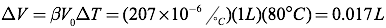# Thermal Expansion

When objects are heated, they tend to expand, and when they are cooled, they tend to contract. You can use this to open glass jars with tight metal lids by running the lids under hot water. The temperature increase in the lid expands the metal lid and the glass jar, but because most metals expand more quickly than glass, the lid becomes looser, making it easier to open the jar.

This occurs because at higher temperatures, objects have higher kinetic energies, so their particles vibrate more. At these higher levels of vibration, the particles aren’t bound to each other as tightly, so the object expands.

The amount an object expands can be calculated for both one-dimensional (linear) and three-dimensional (volumetric) expansion. The amount a material expands is characterized by the material’s coefficient of expansion. In calculating a material’s one-dimensional expansion, you can use the linear expansion formula, and the material’s linear coefficient of expansion (α).When calculating a material’s three-dimensional expansion, you’ll use the volumetric expansion formula, and the material’s volumetric coefficient of expansion (β). Note that in most cases, the volumetric coefficient of expansion is roughly three times the linear coefficient of expansion.The change in temperature in the expansion equations can be given in either degrees Celsius or Kelvins.

A sample table showing coefficients of thermal expansion for selected materials is given below.*Water actually expands when it freezes, so calculations near the freezing point of water require a more detailed analysis than is provided here.Question: A concrete railroad tie has a length 2.45 meters on a hot, sunny, 35°C day. What is the length of the railroad tie in the winter when the temperature dips to -25°C?

Answer: First find the change in the tie’s length.Then, you can find the railroad tie’s final length using the tie’s initial length and its change in length.Question: An aluminum rod has a length of exactly one meter at 300K. How much longer is it when placed in a 400°C oven?

Answer: Since the temperatures are given in two different sets of units, you first need to find the total temperature shift in consistent units (for example, Kelvins).The shift in temperature, therefore, must be 673.15K-300K, or 373.15K. Next, you can use the equation for linear expansion to find the shift in the rod’s length.Question: A glass of water with volume 1 liter is completely filled at 5°C. How much water will spill out of the glass when the temperature is raised to 85°C?

Answer: In this situation, both the glass and the water within will expand as the temperature rises. You can treat both the glass and the water as a volume expansion. Start by finding the expansion of the water.In a similar fashion, you can find the expansion of the glass.The amount of water spilling out is equal to the difference between the water’s expansion and the glass’s expansion, or 0.015 liters.